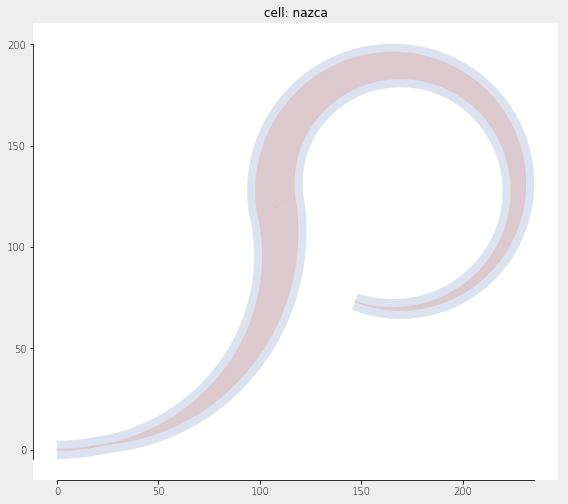Home Forums Nazca Bent Taper 2 Reply To: Bent Taper

#5852

Dear Paul,

There are infinite ways to draw curves (waveguides) between two points. The cobra_p2p is one that specifically does not use a constant bend radius but smoothly goes from a straight waveguide to a curved waveguide and finds an optimal path according to some strategy, as described inside the function.

Hence, the Viper is a good approach to do what you want, but it is not yet an Interconnect. A proper Nazca Interconnect set up has a number of levels to make it work as effortlessly as possible when utilizing it as a designer. It uses a xsection and stores a number of properties that are reused when creating mask_elements, like strt, bend or cobra_p2p.

The following structure gives a short overview on what an Interconnect definition is built upon:

A- xsection:
– add layers and their growth values

– provide a xsection (e.g. get from Interconnect (C))
– provide default values (e.g. get from Interconnect (C))

– – the mask_element is a “closure”, i.e. the function and its environment are saved when creating it.
– – loop over all xsection layers:
– – – apply grow
– – – create and discretize the polygon based on the layer
– – add pins and other properties

C- Interconnect (class):
– add a xsection, see (A)
– add default values (get from xsection where possible)
– Interconnect methods:
– – create an interconnect cell from a mask_element cell (B) and neither cell will be instantiated (by choice).
– – add pins to the interconnect cell

Note that mask_elements exist without Interconnects. In other words, an Interconnect object is just a wrapper around mask_elements to group these elements together inside a single technology definition.

If we now turn to the Viper we find that it creates a spine or path [x(t), y(t)]. From that it creates a polygon by adding a width w(t) to the spine. The viper still needs proper pin directions and polygon geometry at the begin and end points, proper discretization w.r.t. to the mask resolution, loop over all layers in a xsection, etc.

From the user perspective the layout code for a bent waveguide as described in your example simplifies to something as in the example below. It generates the layout shown after the code.
Note that the radius, and width1 and width2, are nicely incorporated as keywords for the tapered_bend() to make it possible overrule defaults.

``````tapered_bend().put(0)
ic.strt().put()
tapered_bend(angle=90, width2=20, N=1000).put()
nd.export_gds()``````The complete code to set it up is shown below. The viper functions x, y, w can be adapted to more or less any sensible set of functions for drawing a waveguide. The example demonstrates part A and B in the above explained structure. Putting it in Interconnect C is straight forward after this step and omitted in this example.

Note that the keywords “anglei” and “angleo” in viper() require Nazca-0.5.8 or up. You can run the code without them in earlier Nazca versions (with the viper) and notice that the viper polygons then do not connect “good enough” in case of a curvature in the begin and/or end point, because the polygon angles are no longer matching the pins their “exact” angle values in those points.

A next step, not in this example, could be to calculate the minimum N for a specific maximum resolution. Here you can take N “large enough”. If this function is called from an Interconnect object you would not use `nd.get_xsection(xs).width` but get the Interconnect’s settings.

``````import numpy as np
import math as m
from functools import partial
import nazca as nd
from nazca.interconnects import Interconnect

def Tp_tapered_bend(x, y, w, radius=100.0, angle=10.0, width1=None, width2=None,
xs=None, layer=None, N=200):
"""Template for a specific Viper implementation.

args:
x (function): function in at least t, t in [0,1]
y (function): function in at least t, t in [0,1]
w (function): function in at least t, t in [0,1]

Returns:
function: Cell generating function
"""

"""Specific Viper implementation.

Returns:
Cell: Cell based on a Viper
"""
N = N # discretization steps should be "large enough" for mask resolution
name = 'viper_bend'

# housekeeping for default values (add as needed):
if width1 is None:
width1 = nd.get_xsection(xs).width
if width2 is None:
width2 = nd.get_xsection(xs).width

# Fill in all x, y, w function parameters except t:
W = partial(w, width1=width1, width2=width2)

# Store begin and end points of the viper.
# Note-1: begin and end angle of the viper spine should be taken
#     infinitesimally close to the begin and end.
# Note-2: the polygon's begin and end edge should be perpendicular
#     to the local angles from Note-1
xa, ya = X(0), Y(0)
xb, yb = X(1), Y(1)
d = 1e-8
aa = m.degrees(m.atan2( Y(0)-Y(d), X(0)-X(d)))
ab = m.degrees(m.atan2( Y(1)-Y(1-d), X(1)-X(1-d)))
AB = (ab-aa-90)
if AB < -180:
AB += 180

# create the Cell:
with nd.Cell(name=name, cnt=True) as C:
for lay, grow, acc, line in nd.layeriter(xs, layer):
(a1, b1), (a2, b2), c1, c2 = grow
xygon = nd.util.viper(
X,
Y,
lambda t: W(t) + b1-b2, # add the layer growth
N=N,
anglei = -aa, # remove for <0.5.8
angleo = AB   # remove for <0.5.8
)
nd.Polygon(points=xygon, layer=lay).put(0)
nd.Pin(name='a0', type=0, width=width1, xs=xs, show=True).put(xa, ya, aa)
nd.Pin(name='b0', type=1, width=width2, xs=xs, show=True).put(xb, yb, ab)
nd.put_stub(length=0)
return C
return tapered_bend

if __name__ == "__main__":
XS.width = 1.0
ic = Interconnect(xs='xs')

# create a specific viper-based mask_element:
return radius * np.cos(t * angle/180 * np.pi)